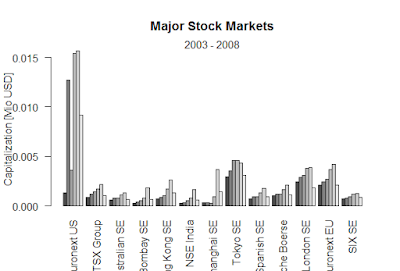Showing posts with label stockdatascience. Show all posts
Showing posts with label stockdatascience. Show all posts

## How to find Higher-Order Moments stock data science

"AAPL" "CSCO"

tail(stkdata)
2020-01-16        315.24         49.05
2020-01-17        318.73         49.02
2020-01-21        316.57         48.80
2020-01-22        317.70         49.07
2020-01-23        319.23         49.00
2020-01-24        318.31         48.85
> aapl=AAPL[’2007−01−03::2020−01−17’]
Error: unexpected input in "aapl=AAPL[’"
>
> aapl=AAPL['2007−01−03::2020−01−17']
> csco =CSCO['2007−01−03::2020−01−17']
> aapl = as.matrix(AAPL[ , 6 ])
> csco = as.matrix(CSCO[ , 6 ])
> stkdata = cbind (aapl,csco)
>
> dim(stkdata)
 3288    2
>
> n = length(stkdata[,1])
> n
 3288
> rets = log( stkdata [ 2 : n , ]/stkdata[1:( n−1) ,])

### > MEAN

> colMeans(rets)
0.0010403556  0.0002501597
> cv = cov ( rets )
>
> print( cv , 2 )
>
> cr = cor( rets )
>
> print ( cr,2 )
x = matrix(rnorm ( 4 ) , 2 , 2 )

> x
[,1]     [,2]
[1,]  0.512371 1.176699

[2,] -2.124443 0.676594
> print( t( x ) , 2 )
[,1]  [,2]
[1,] 0.51 -2.12

[2,] 1.18  0.68
> print ( t( x ) %*% x , 2 )
[,1]  [,2]
[1,]  4.78 -0.83
[2,] -0.83  1.84

> print ( x %*% t ( x ) , 2 )
[,1]  [,2]
[1,]  1.65 -0.29

[2,] -0.29  4.97
> cv_inv = solve( cv )

> print ( cv_inv , 2 )

> print ( cv_inv %*% cv , 2 )

> library("corpcor", lib.loc="~/R/win-library/3.6")
> is.positive.definite( cv )
 TRUE
> is.positive.definite(x)
 FALSE
> is.positive.definite( x %*% t( x ))

 TRUE
Stock data in place, and we can compute daily

returns, and then convert those returns into annualized returns.
> rets_annual = rets*252
> print (c(mean( rets ) ,mean( rets_annual)))

 0.0006452577     0.1626049311
Compute the daily and annualized standard deviation of returns
> r_sd = sd( rets )
>
> r_sd_annual = r_sd*sqrt ( 252 )
> print(r_sd_annual)
 0.2999346
> print(c( r_sd, r_sd_annual))
 0.0188941 0.2999346
>
> print(sd (rets*252))
 4.761314
>
> print(sd (rets*252))/252
 4.761314
 0.0188941
> print( sd(rets*252))/sqrt(252 )
 4.761314
 0.2999346
The variance is easy as well.
> r_var = var( rets )

> r_var_annual = var ( rets )*252
> print ( c( r_var , r_var_annual ) )
 0.0003869694 0.0001751147 0.0001751147
 0.0003268013 0.0975162890 0.0441289039

 0.0441289039 0.0823539370
Higher-Order Moments
Skewness means one tail is fatter than the other (asymmetry). Fatter right (left) tail implies positive (negative) skewness.
> skewness ( rets )
-0.4691675     -0.5079582
Kurtosis means both tails are fatter than with a normal
distribution.
> kurtosis (rets)

10.22829      14.64755
Example-For the normal distribution, skewness is zero, and kurtosis is 3. Kurtosis
minus three is denoted “excess kurtosis
> skewness(rnorm(1000000))
 -0.0003906446

> kurtosis(rnorm (1000000))
 3.007031
Using properties of the lognormal distribution, the conditional mean of the stock price becomes E[S(t + h)|S(t)] = S(t) · e^µh

#### Annualized volatility σ

> h = 1/252
> sigma = sd( rets)/sqrt(h)
> sigma
 0.2999346
The parameter µ is also easily estimated as
> mu = mean(rets)/h+0.5*sigma^2
> mu
 0.2075853.

### Black-Scholes formula-R

Black-Scholes formula-R > BlackScholes <- function(TypeFlag = c("c", "p"), S, X, Time, r, b, sigma) { TypeFla...